Latest Banking jobs   »

# Quantitative Aptitude Quiz For IDBI AM/ Bank of India PO 2023-14th March

Direction (1 – 5): In the following questions, two equations, I and II are given. You have to solve both the equations and give answer accordingly.
(a) if x>y
(b) if x ≥ y
(c) if x<y
(d) if x ≤ y
(e) if x=y or no relation.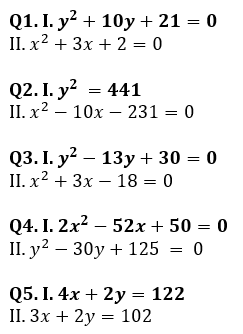Directions (6-10): In each of these questions, two equations (I) and (II) are given. You have to solve both the equations and give answer
(a) if x>y
(b) if x≥y
(c) if x = y or no relation can be established between x and y.
(d) if y>x
(e) if y≥x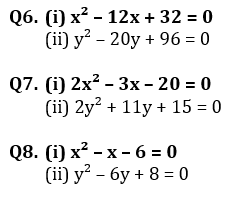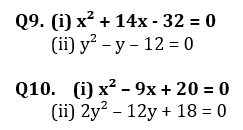Directions (11-15): In each of these questions, two equations (I) and (II) are given. You have to solve both the equations and give answer
(a) if x>y
(b) if x≥y
(c) if x = y or no relation can be established between x and y.
(d) if y>x
(e) if y≥x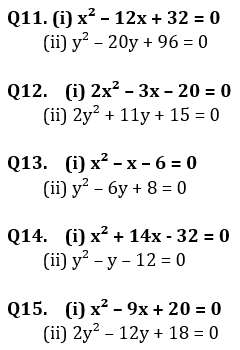Solutions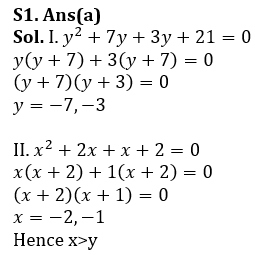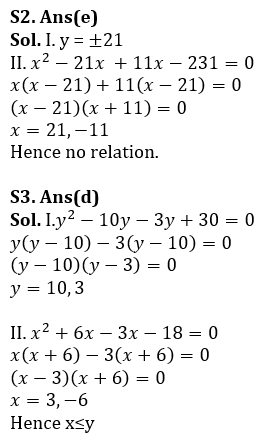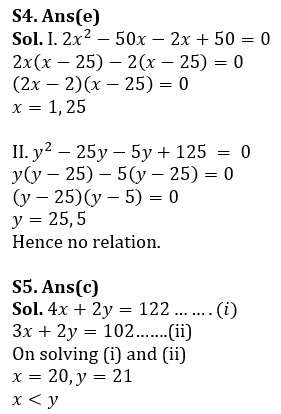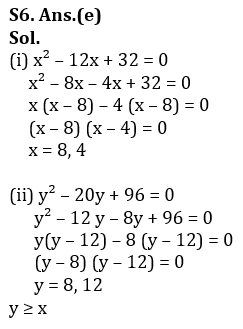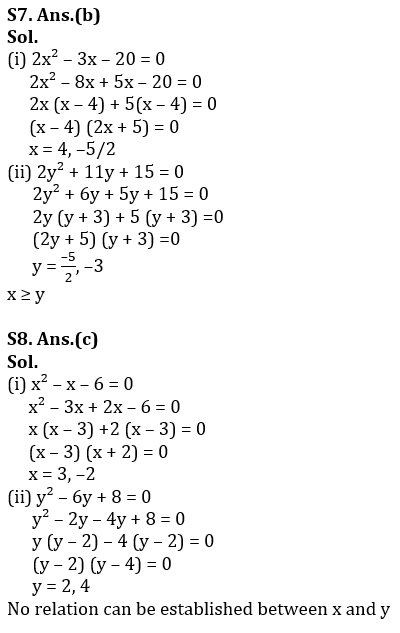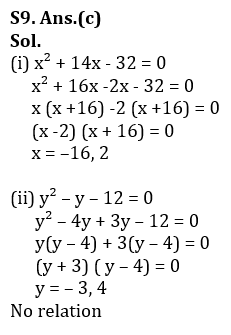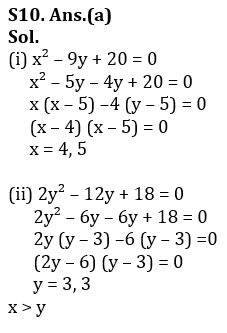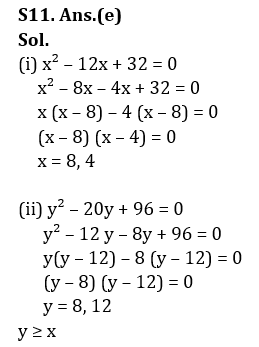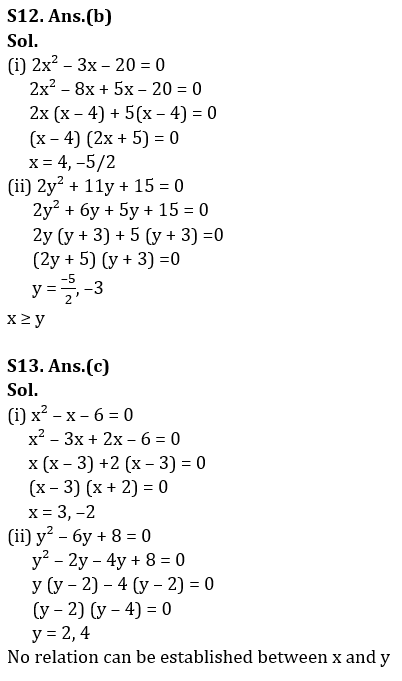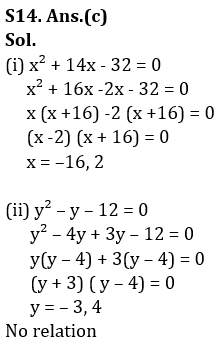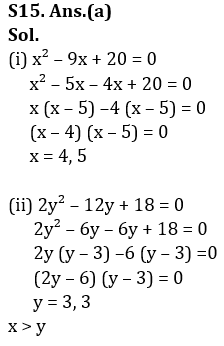.

## FAQs

### What is the selection process of the IDBI AM?

The selection process of IDBI AM is Online exam and Interview.

#### Congratulations!Union Budget 2023-24: Free PDF